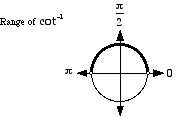# inverse cotangent

Inverse Cotangent

 cot-1 ctg-1 Cot-1 Ctg-1 arccot arcctg Arccot Arcctg

Basic idea: To find cot-1 1, we ask "what angle has cotangent equal to 1?" The answer is 45°. As a result we say cot-1 1 = 45°. In radians this is cot-1 1 = π/4.

More: There are actually many angles that have cotangent equal to 1. We are really asking "what is the simplest, most basic angle that has cotangent equal to 1?" As before, the answer is 45°. Thus cot-1 1 = 45° or cot-1 1 = π/4.

Details: What is cot-1 (–1)? Do we choose 135°, –45°, 315°, or some other angle? The answer is 135°. With inverse cotangent, we select the angle on the top half of the unit circle. Thus cot-1 (–1) = 135° or cot-1 (–1) = 3π/4.

In other words, the range of cot-1 is defined to be the angles on the upper half of the unit circle as pictured below. The range of cot-1 is restricted to (0, 180°) or (0, π).

Note: arccot refers to "arc cotangent", or the radian measure of the arc on a circle corresponding to a given value of cotangent.

Technical note: Since none of the six trig functionssine, cosine, tangent, cosecant, secant, and cotangent are one-to-one, their inverses are not functions. Each trig function can have its domain restricted, however, in order to make its inverse a function. Some mathematicians write these restricted trig functions and their inverses with an initial capital letter (e.g. Cot or Cot-1). However, most mathematicians do not follow this practice. This website does not distinguish between capitalized and uncapitalized trig functions.